# 3 Descriptives and visualizations

## 3.1 Overview

In this section, I describe and visualize the variables. We got variables on the person-level and on the wave level.

Person-level

• Age and gender (`age`, `gender`)
• What region of the UK participants are from (`region`)
• Whether they’ve used a medium in the three months before the first wave (variables starting with `filter`)
• To what extent they identify with a medium (variables ending with `identity`)

Wave-level

• How much they used a medium per wave (variables ending with `time`)
• Their well-being per wave (`well_being`)

## 3.2 Person-level

Let’s have a look at the final sample. Overall, our sample size is N = 2159. Participants, on average, were M = 47 years old, SD = 14.6, see Figure 3.1. The gender distribution is pretty equal (1123 women (52.01%), 1035 men, and 1 non-binary participants).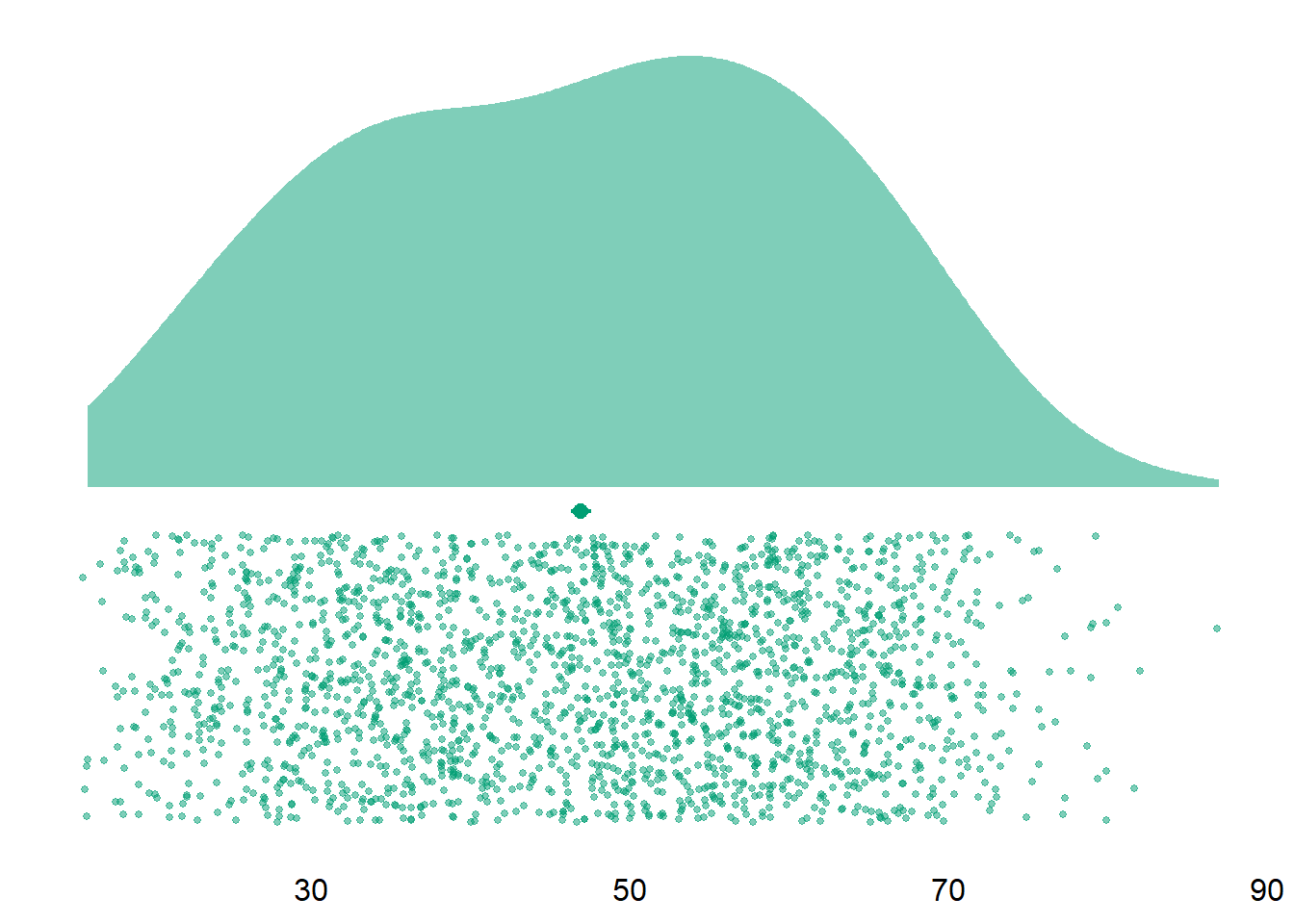Figure 3.1: Distribution of age

Alright, next let’s have a look again (now that we have the final sample) at how many people had used a medium in the three months before wave 1 (Table 3.1).
Table 3.1: Frequency of how much a medium was used at wave 1
Medium Used Not Used Proportion
audiobooks 274 1885 12.7
ebooks 1032 1127 47.8
films 1339 820 62.0
magazines 498 1661 23.1
music 1420 739 65.8
tv 1310 849 60.7
video_games 624 1535 28.9
Let’s inspect the sample characteristics (age and gender) by how many surveys a person filled out (Table 3.2). So we’re just looking at whether there are pronounced differences in sample charateristics as people dropped out of the study. Looks pretty stable to me, especially given the samller sample sizes for those who dropped out early.
Table 3.2: Demographics by number of filled out surveys
Waves N Mean age SD age Women Men Other Proportion women (%)
1 982 40.64766 17.66912 448 531 3 46
2 402 42.98259 15.97183 192 210 0 48
3 219 43.27854 16.47406 113 106 0 52
4 174 44.81609 16.11414 99 75 0 57
5 831 48.41516 13.63305 435 395 1 52
6 1022 46.15753 14.63435 515 506 1 50
Now let’s have a look at how much people identified with each medium. Note that people weren’t asked those identity questions if they indicated that they hadn’t used a medium at wave 1.
Table 3.3: Descriptive information for identity scales
variable N mean sd median min max range cilow cihigh
music_identity 1420 2.19 0.67 2.14 1 4 3 2.16 2.23
tv_identity 1310 2.16 0.63 2.14 1 4 3 2.13 2.20
films_identity 1339 2.26 0.67 2.14 1 4 3 2.22 2.29
games_identity 624 2.48 0.82 2.43 1 4 3 2.42 2.55
e_publishing_identity 1211 2.33 0.69 2.29 1 4 3 2.29 2.37
Figure 3.2 shows the distributions of those identity variables. Apparently gamers identified more with games than for example music listeners identified with music.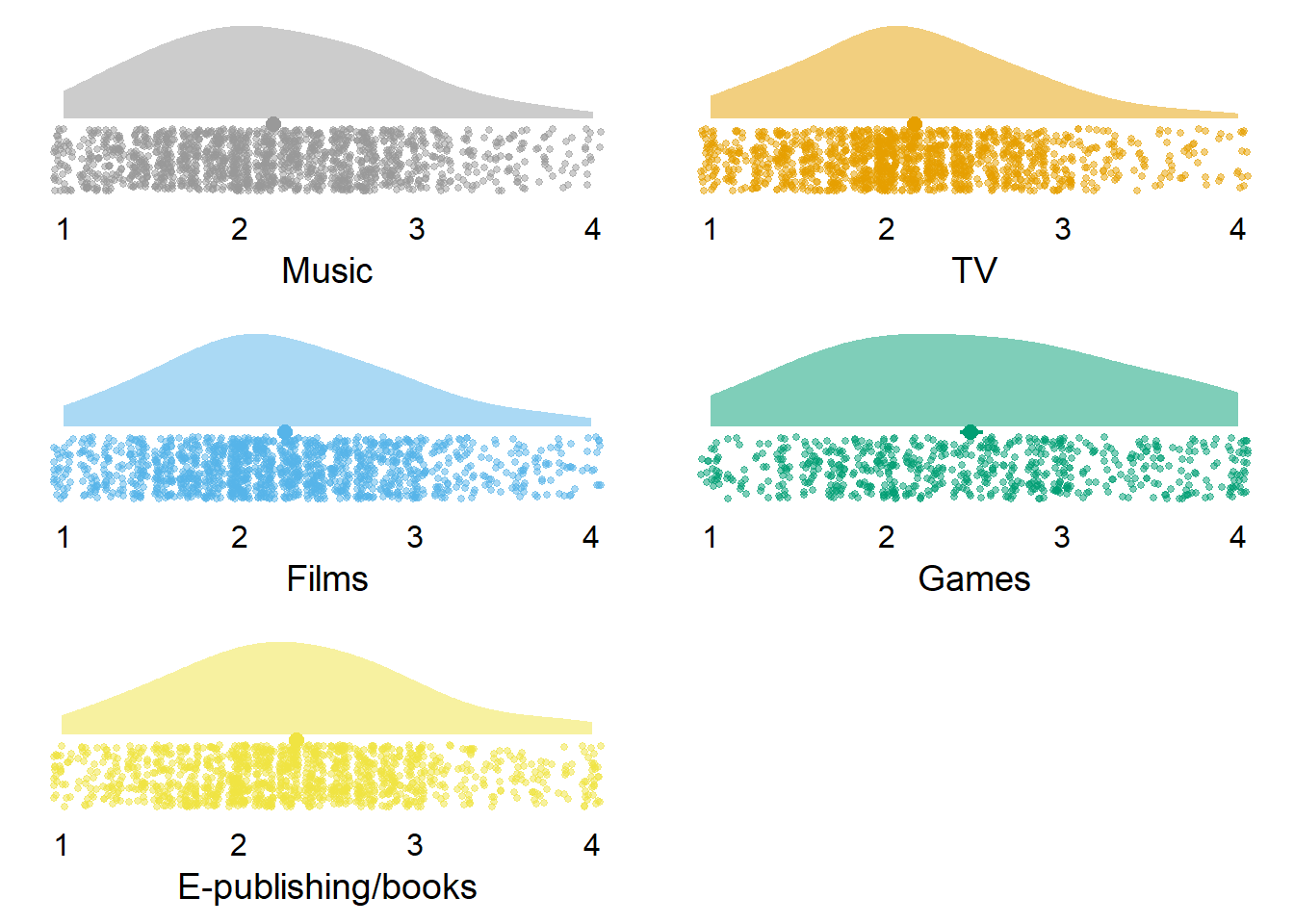Figure 3.2: Distribution of identity variables

## 3.3 Wave-level

Let’s inspect the descriptive information for the different media. Table 3.4 shows that people used TV, music, and films the most, but spent little time on magazines and audio books.
Table 3.4: Descriptive information for use time (including zero estimates)
variable N mean sd median min max range cilow cihigh
music_time 10985 2.28 2.57 2.00 0 22.22 22.22 2.24 2.33
tv_time 10985 3.25 3.38 2.50 0 22.00 22.00 3.18 3.31
films_time 10985 2.33 2.62 2.00 0 22.25 22.25 2.28 2.37
games_time 10985 0.98 2.24 0.00 0 22.00 22.00 0.94 1.02
books_time 10985 1.09 1.96 0.17 0 21.00 21.00 1.05 1.12
magazines_time 10985 0.24 0.87 0.00 0 20.00 20.00 0.23 0.26
audiobooks_time 10985 0.16 0.78 0.00 0 20.00 20.00 0.15 0.18
Figure 3.3 shows the distributions of those time variables. Note that most distributions are zero-inflated with a heavy skew.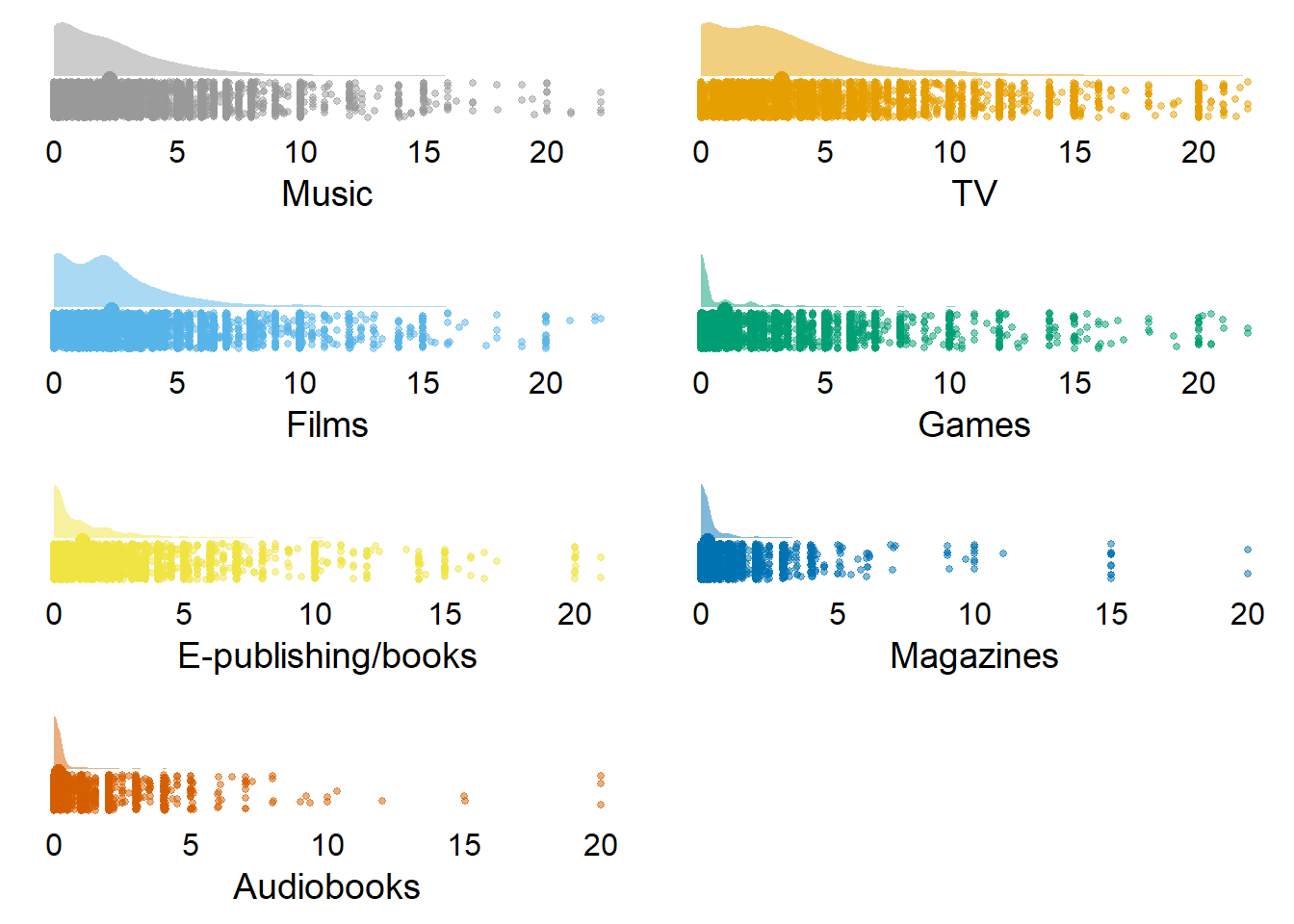Figure 3.3: Distribution of time variables (with zeros)

Let’s also inspect those summary statistics and distributions without zeros. Table 3.5 shows the same pattern was with zeros, but overall higher means. Interestingly, those who listen to audiobooks report to do so for a long time per day.
Table 3.5: Descriptive information for use time (excluding zero estimates)
variable N mean sd median min max range cilow cihigh
music_time 8251 3.04 2.54 2.25 0.02 22.22 22.20 2.99 3.09
tv_time 8537 4.18 3.29 3.00 0.02 22.00 21.98 4.11 4.25
films_time 7956 3.21 2.58 2.33 0.02 22.25 22.23 3.15 3.27
games_time 3873 2.78 3.04 2.00 0.02 22.00 21.98 2.69 2.88
books_time 5561 2.14 2.31 1.50 0.02 21.00 20.98 2.08 2.21
magazines_time 2442 1.09 1.58 0.67 0.02 20.00 19.98 1.03 1.16
audiobooks_time 1101 1.64 1.93 1.00 0.02 20.00 19.98 1.52 1.75
Figure 3.4 shows a less skewed distribution once we remove zeros.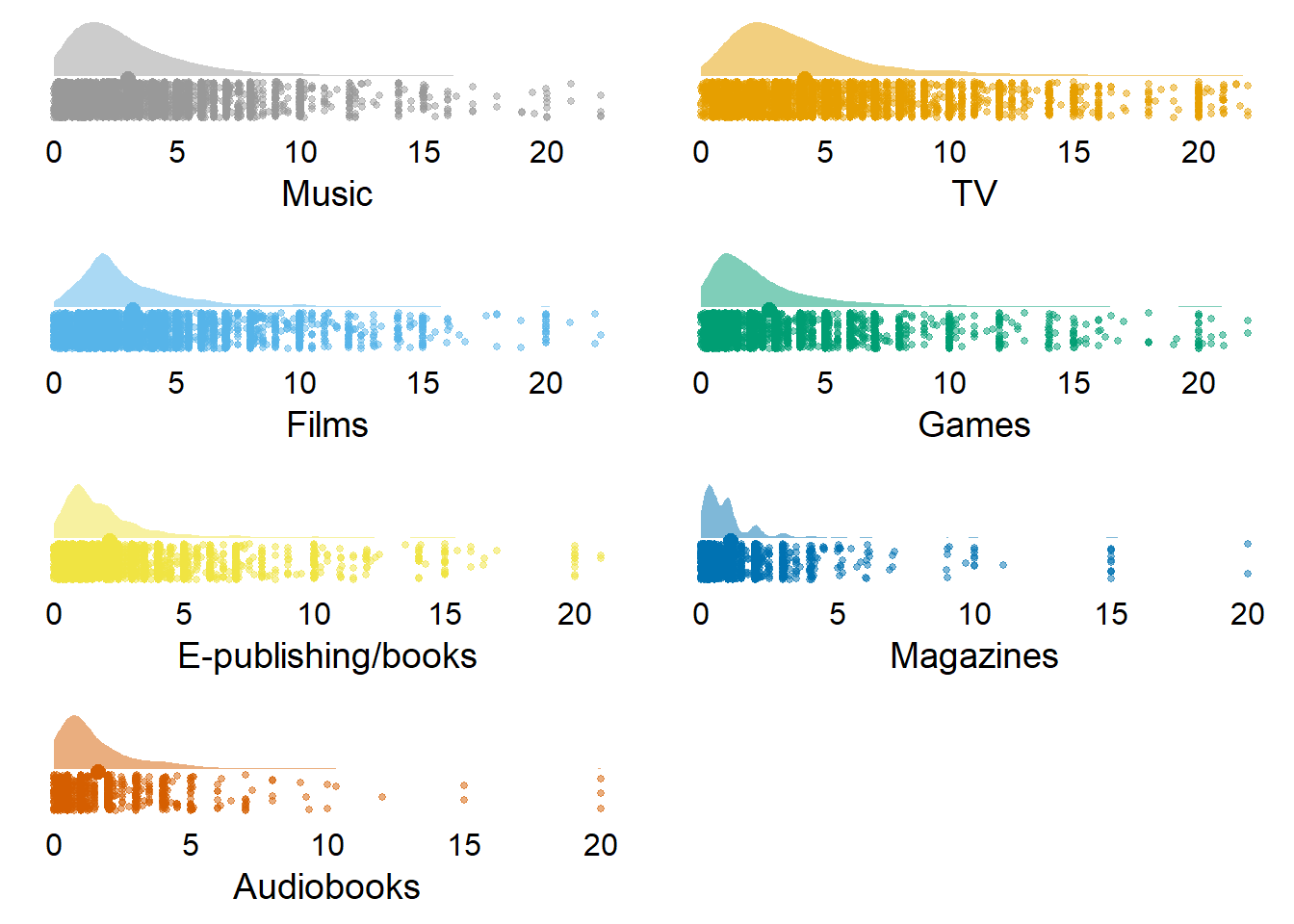Figure 3.4: Distribution of time variables (without zeros)

As a next step, let’s see how use developed over time. Figure 3.5 shows that most media stay stable, except maybe an overall spike in wave 2.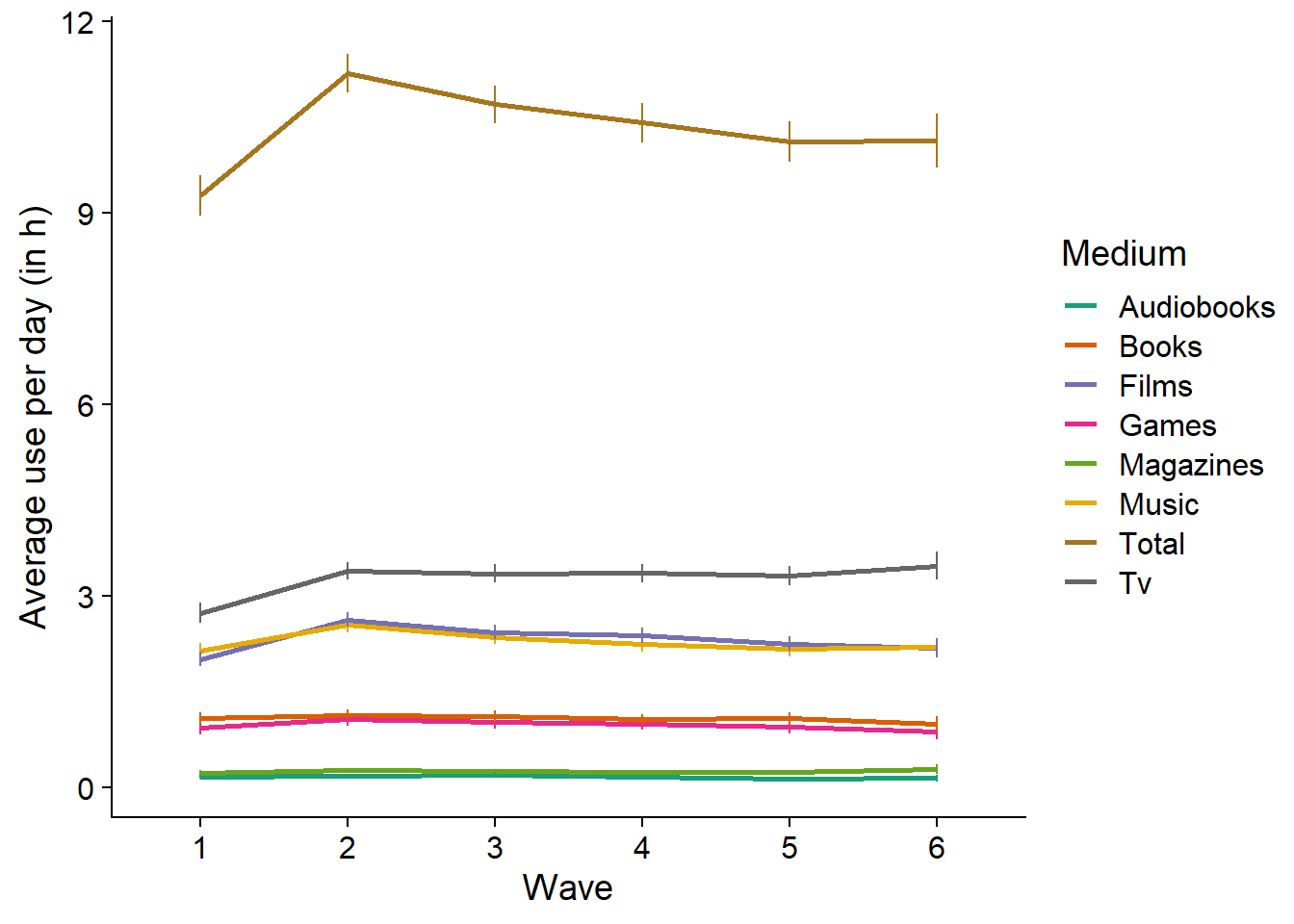Figure 3.5: Average use over time per medium

Last, let’s see the overall descriptives of the well-being variables. Table 3.6 shows that both life satisfaction and affect were above the mid-point of the scale - even though for affect, it’s a close call.
Table 3.6: Well-being descriptives
variable N mean sd median min max range cilow cihigh
affect 10985 5.94 2.18 6 0 10 10 5.90 5.98
life_satisfaction 10985 6.40 2.08 7 0 10 10 6.37 6.44
Let’s plot the distribution of both affect and life satisfaction in Figure 3.6.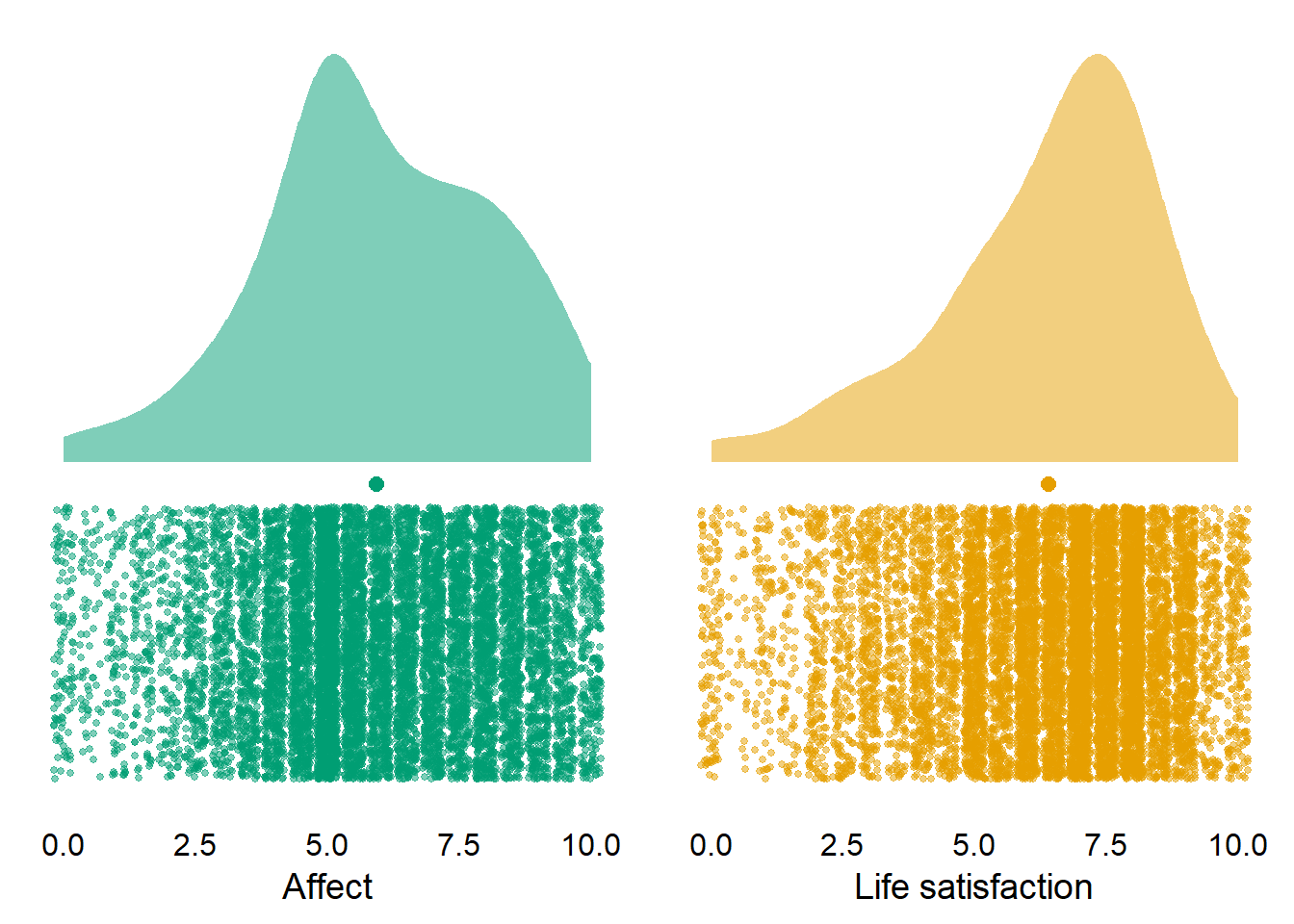Figure 3.6: Distribution of well-being across waves and participants

Last, let’s look at how well-being develops over time. Figure 3.7 shows that life satisfaction appears stable: note the y-axis is on decimal points (original range: 0 to 10). In contrast, affect has significantly decreased, mostly because anxiety has decreased (not shown on graph).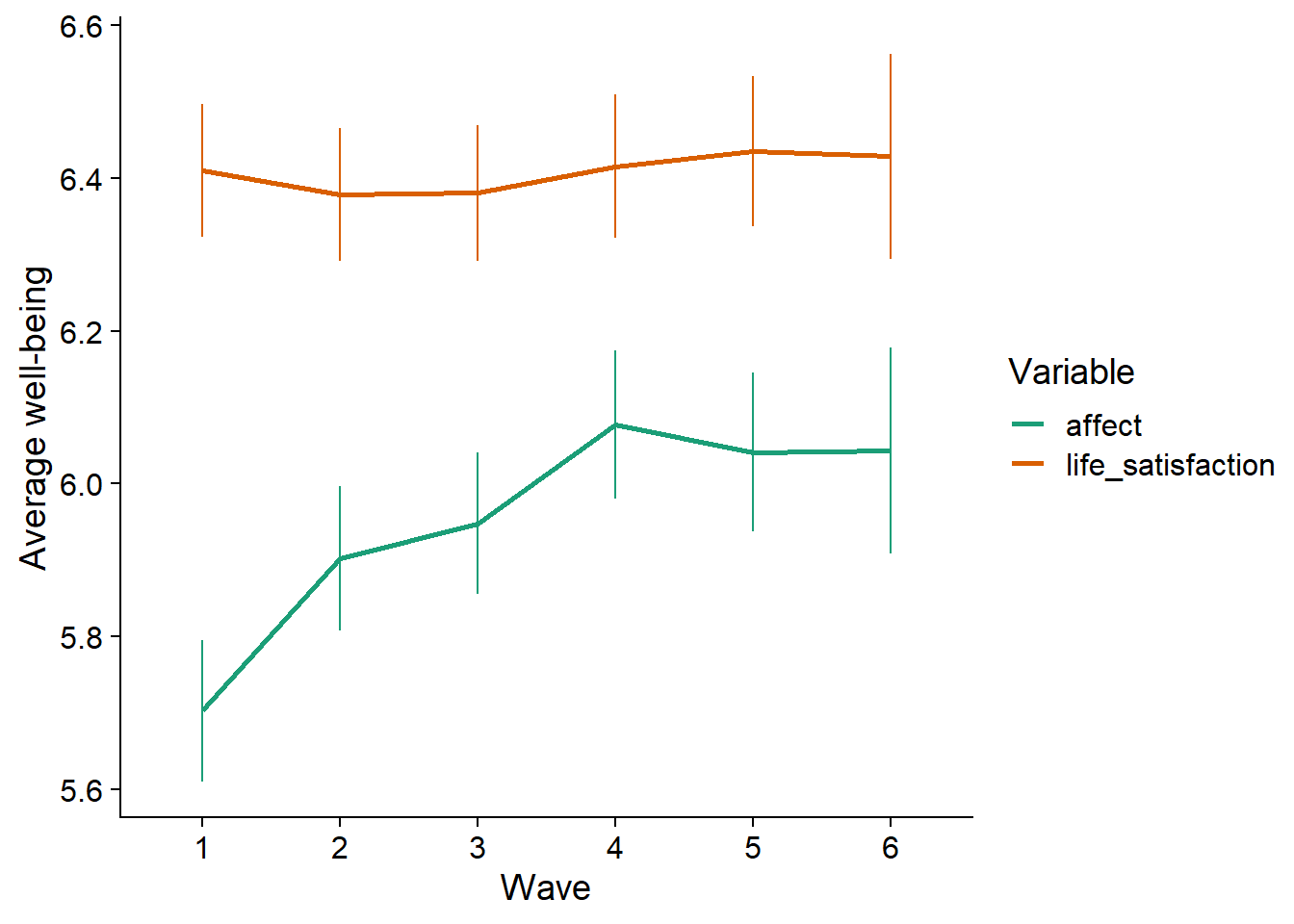Figure 3.7: Average estimates over time per medium

## 3.4 Correlation matrices

Let’s have a look at the row correlations between the media use variables and well-being. Figure 3.8 shows that a) most media use is correlated, and b) there are negative, but very small correlations between well-being and media use. The significance is negligible here just because the sample is so large. That said, we haven’t done any grouping yet, so the correlation matrix treats all observations as independent, which is clearly not the case.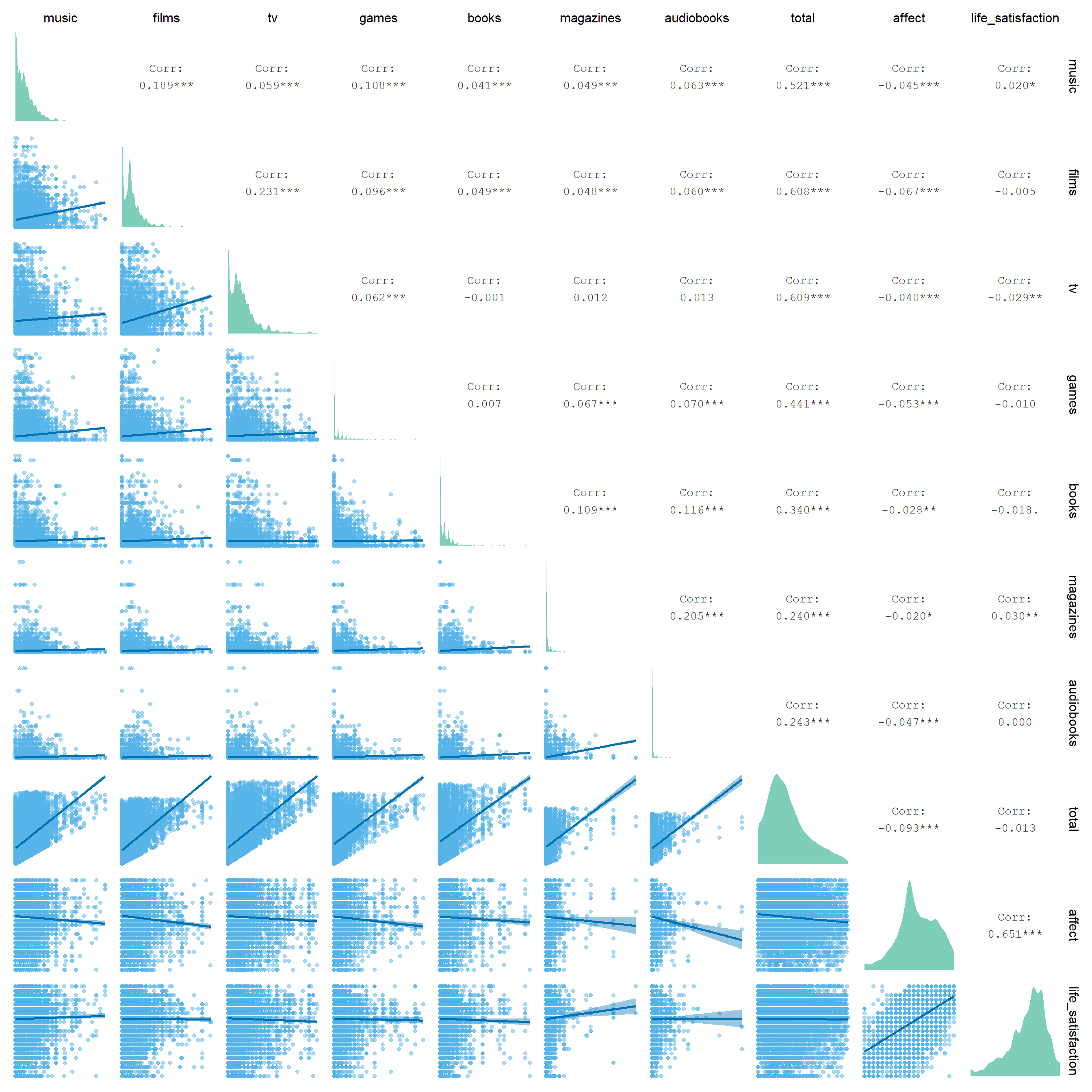Figure 3.8: Correlation matrix of hours spent with a medium and well-being

At the request of a reviewer, we can also look at correlations per age group. We leave a formal analysis up to readers, who can estimate models with an interaction term. Here, I merely plot the slope between total media time and well-being by age groups. In Figure 3.9, we see that there are differences in age on well-being, but not in slopes.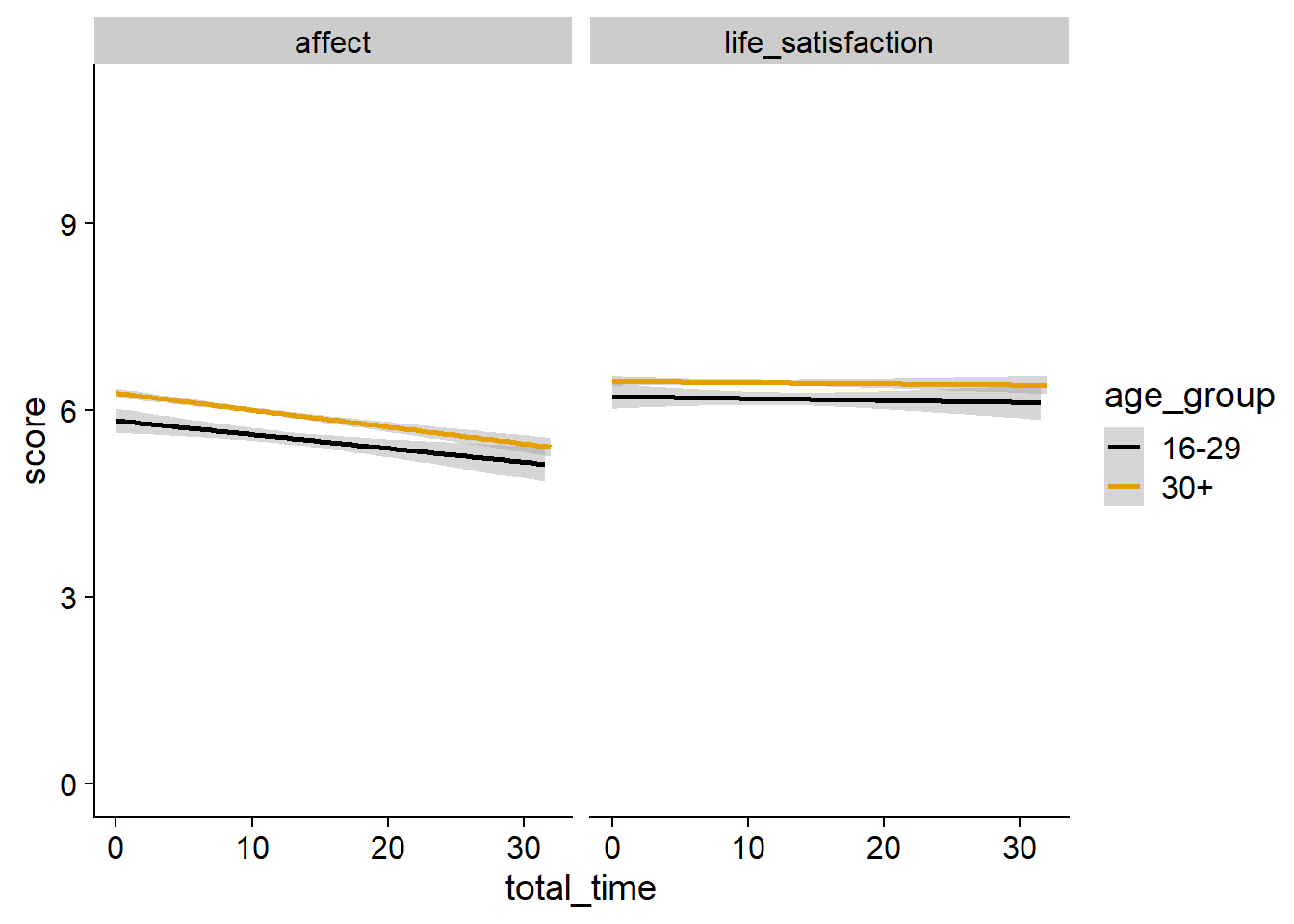Figure 3.9: Relation between total time and well-being by age

The reviewer was also interested in different lags. However, to see each correlation at each wave with each lag for each medium would be 6 (lags medium) x 6 (lags well-being) x 2 (well-being measures) x 7 (media types) = 504 correlations. Therefore, Figure 3.10 shows the correlations of total time with all media with affect at the last wave for total time at each wave. We see little differences in the slopes, meaning that a different lag for the analysis would probably not change the results much. However, we invite readers to run the analysis themselves with a different lag.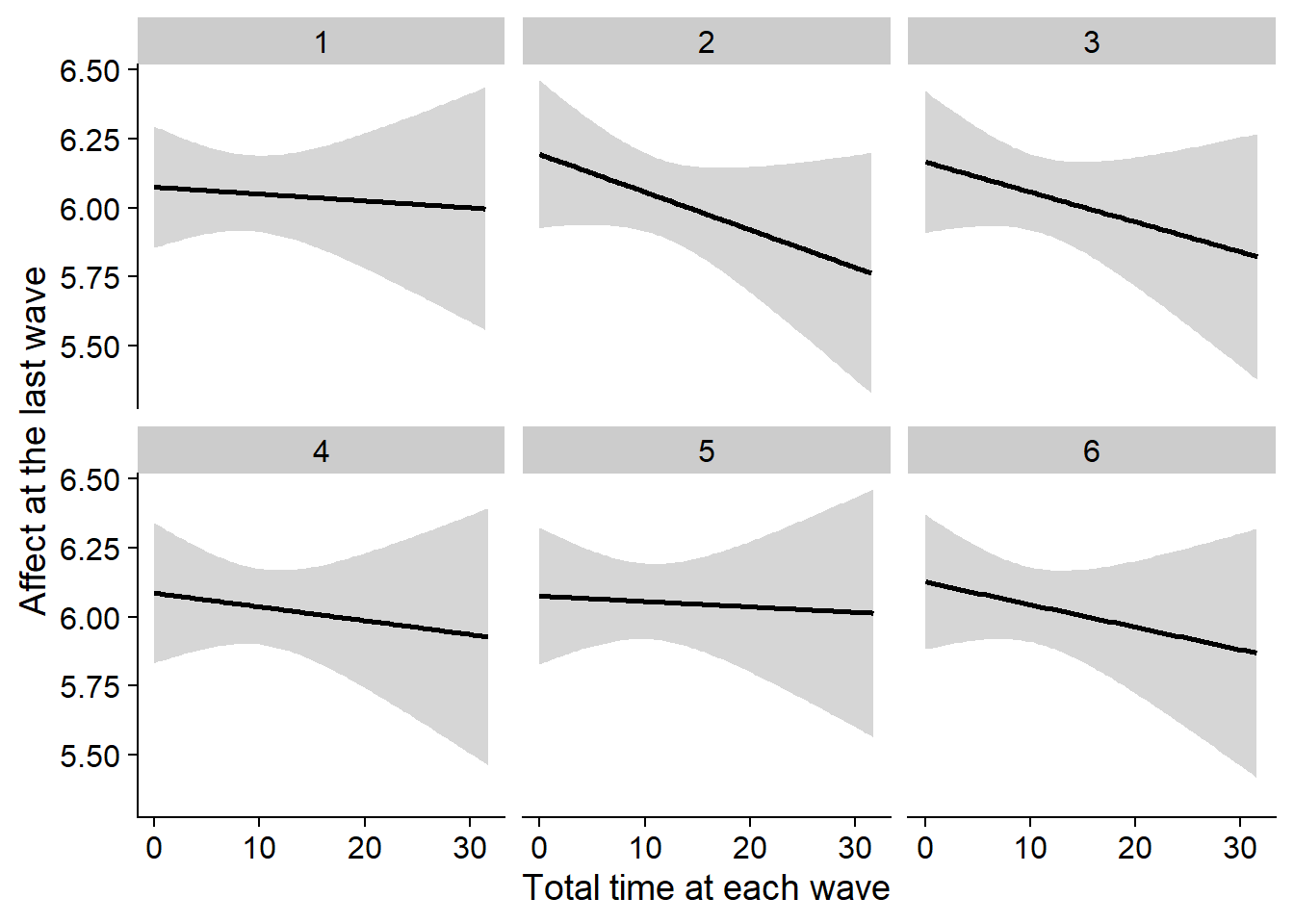Figure 3.10: Correlation between affect at the last wave and total time at each wave

## 3.5 Plots for Paper

For the paper, I want to avoid confronting readers with blocks of numbers. Therefore, I’ll try to put as much info as possible into figures rather than tables or in-text reports of numbers.

I think the following plots are necessary for the paper:

1. A plot of the response rate per wave, simply as a descriptive measure.
2. A plot showcasing the exclusions. I’ll describe the exclusion criteria in the paper, but don’t want to overwhelm readers with numbers for each.
3. A plot showing the distributions, M, and SD of media use for different categories.
4. Same plot, but for well-being.
5. A plot of the results.

First, a plot for the response rate for each wave. We already showed response rates as a table in the processing section, so we can re-use the `completion_table` object for a plot. Not sure about the colors yet, might go all black for this one.

``````ggplot(
data = completion_table %>%
rename(
Wave = Waves,
`Participants per wave (%)` = `Participants per wave`
) %>%
mutate(
Wave = as.factor(Wave)
),
aes(
x = Wave,
y = `Participants per wave (%)`#,
# color = Wave,
# fill = Wave
)
) +
geom_segment(
aes(
xend = Wave,
y = 0,
yend = `Participants per wave (%)`
),
size = 1
) +
geom_point(
size = 2
) +
geom_text(
aes(
label = paste0(`Participants per wave (%)`, "(", `Frequency per wave`, "%)"),
y = `Participants per wave (%)` + 160
)
) +
scale_colour_manual(values=cb_palette) +
scale_fill_manual(values = cb_palette) +
theme(
legend.position = "none"
) -> figure1

figure1``````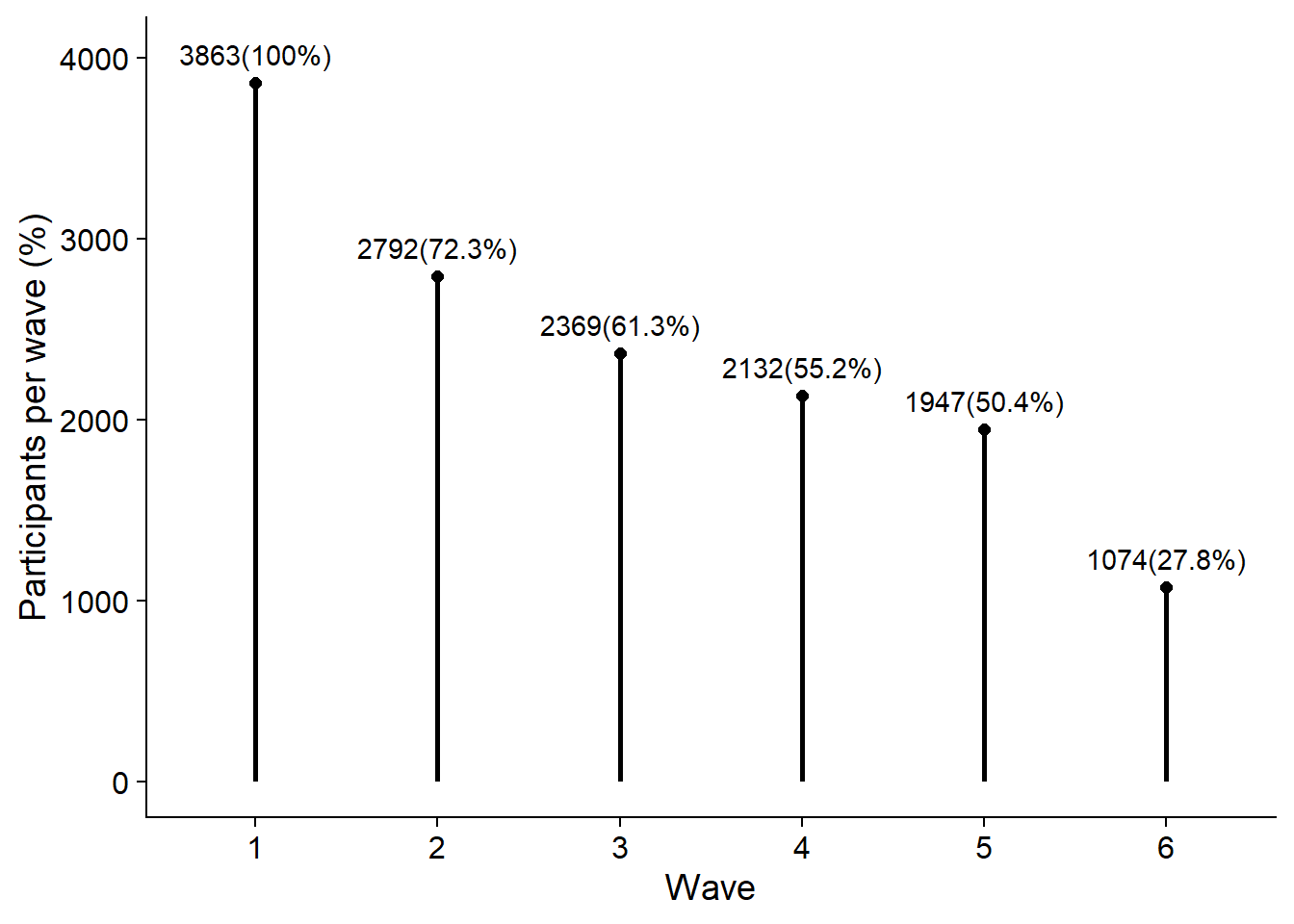``````# create figure folder
dir.create("figures/", FALSE, TRUE)

ggsave(
filename = here("figures", "figure1.png"),
plot = figure1,
width = 21 * 0.8,
height = 29.7 * 0.4,
units = "cm",
dpi = 300
)``````

Next, I plot the exclusions. There’s two ways to go about this. Either I show the absolute number of participants and observations that we exclude with each criterion in relation to the total sample size. I do that below. Note that getting those exclusions in a nice format requires some wrangling. I already created the `exclusion_plot_data` object in the processing section. Here, I turn it into the long format first.

``````# to long format for plotting
exclusion_plot_data <-
exclusion_plot_data %>%
filter(!str_detect(Exclusion, "Total")) %>% # don't include the total numbers for now
rename(Participants = PPs) %>% # nicer name
pivot_longer(
Participants:Observations,
names_to = "Measure",
values_to = "Value"
) %>%
mutate( # create new variable that's stable across exclusions
Total = case_when(
Measure == "Participants" ~ exclusion_plot_data %>% filter(Type == "Total") %>% pull(PPs),
TRUE ~ exclusion_plot_data %>% filter(Type == "Total") %>% pull(Observations),
),
`Total Before` = case_when(
Measure == "Participants" ~ exclusion_plot_data %>% filter(Type == "Total Before") %>% pull(PPs),
TRUE ~ exclusion_plot_data %>% filter(Type == "Total Before") %>% pull(Observations),
)
) %>%
mutate(
across(
Type:Measure,
as.factor
)
)

# https://stackoverflow.com/questions/11889625/annotating-text-on-individual-facet-in-ggplot2
# I want to show the total numbers once, not for each facet/criterion combination, which is why I create a data frame that only has a matching value for the positions in the plot that I want
label_positions <-
tibble(
Type = rep("Wave-level", 2),
Exclusion = c(3, 3), # on the right, where wave-level exclusions are
Measure = c("Participants", "Observations")
) %>%
mutate(
across(
everything(),
as.factor
)
)

label_positions <-
left_join(
label_positions,
exclusion_plot_data %>% select(-Value)
)

# then plot
ggplot(
exclusion_plot_data,
aes(
x = Exclusion,
y = Value,
color = Type
)
) +
geom_segment(
aes(
x = Exclusion,
xend = Exclusion,
y = 0,
yend = Value
),
alpha = 1,
size = 1
) +
facet_grid(Measure ~ Type, scales = "free") +
geom_point(alpha = 1, size = 2) +
geom_text(
aes(
label = paste0("-", `Total Before` - Value),
x = as.numeric(Exclusion) + 0.35,
y = Total * 0.1
)
) +
geom_hline(
aes(
yintercept = Total
),
linetype = "dashed"
) +
geom_hline(
aes(
yintercept = `Total Before`
),
linetype = "solid"
) +
geom_text(
data = label_positions,
aes(
label = `Total`,
x = 3.3,
y = Total * 1.05
),
color = "black"
) +
geom_text(
data = label_positions,
aes(
label = `Total Before`,
x = 3.3,
y = `Total Before` * 1.05
),
color = "black"
) +
xlab("Exclusion Criterion") +
scale_colour_manual(values=cb_palette) +
scale_fill_manual(values = cb_palette) +
theme(
axis.title.y = element_blank(),
legend.position = "none",
panel.background=element_blank(),
panel.border=element_blank(),
panel.grid.major=element_blank(),
panel.grid.minor=element_blank(),
plot.background=element_blank(),
strip.background = element_blank()
) -> exclusion_figure1

exclusion_figure1``````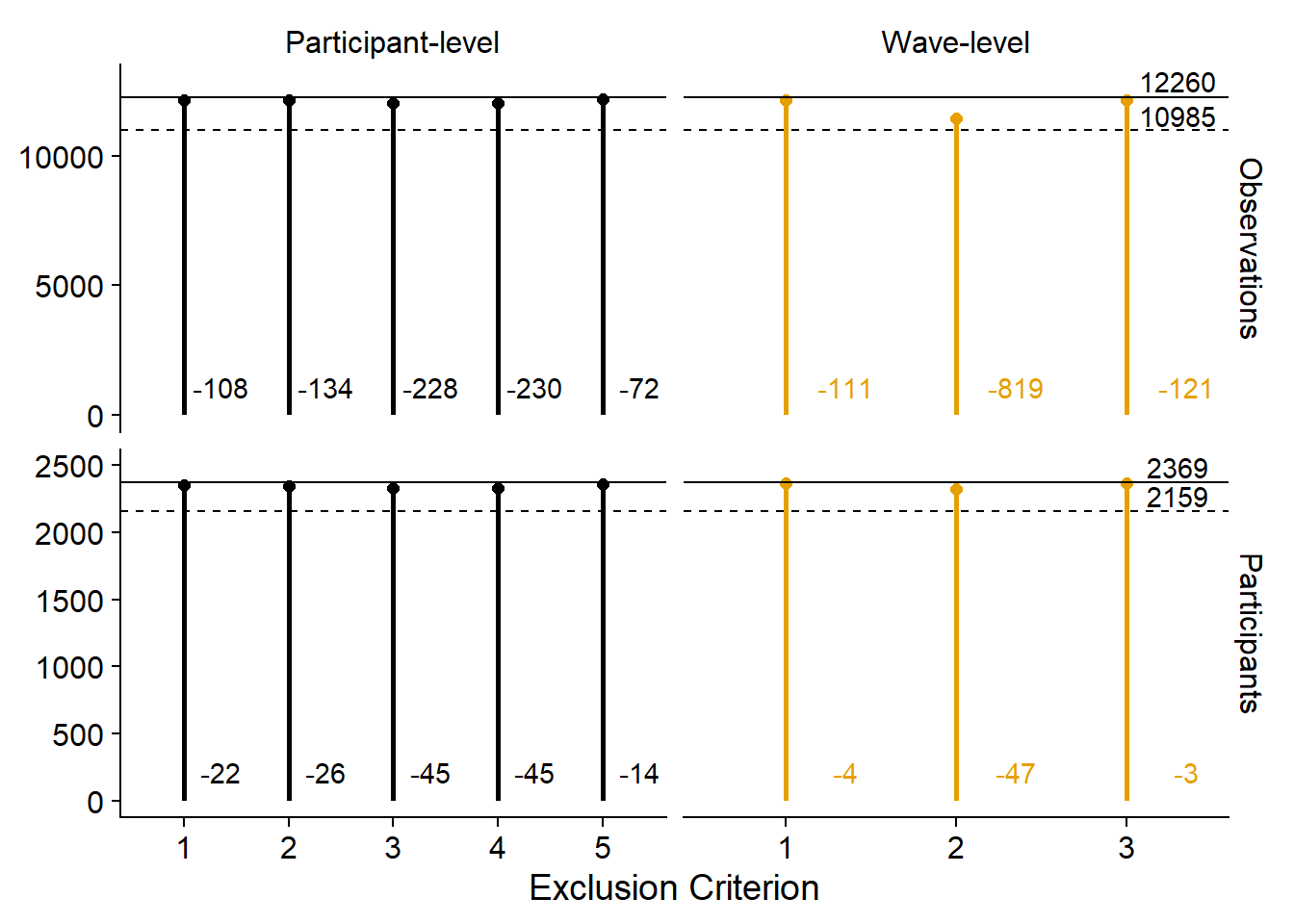Alternatively, we could emphasize the contributions of each criterion in relation to the other criteria. Not sure I like this because it doesn’t show the total sample size and the reduction in total sample size. Then again, could simply add those to the figure captions.

``````ggplot(
exclusion_plot_data %>%
mutate(
Value = `Total Before` - Value
),
aes(
x = Exclusion,
y = Value,
color = Type
)
) +
geom_segment(
aes(
x = Exclusion,
xend = Exclusion,
y = 0,
yend = Value
),
alpha = 1,
size = 1
) +
facet_grid(Measure ~ Type, scales = "free") +
geom_point(alpha = 1, size = 2) +
geom_text(
aes(
label = paste0(round(Value/`Total Before`, digits = 4) * 100, "%"),
y = Value
),
vjust = 1,
hjust = -0.2,
size = 3
) +
xlab("Exclusion Criterion") +
scale_colour_manual(values=cb_palette) +
scale_fill_manual(values = cb_palette) +
theme(
axis.title.y = element_blank(),
legend.position = "none",
panel.background=element_blank(),
panel.border=element_blank(),
panel.grid.major=element_blank(),
panel.grid.minor=element_blank(),
plot.background=element_blank(),
strip.background = element_blank()
) -> exclusion_figure2

exclusion_figure2``````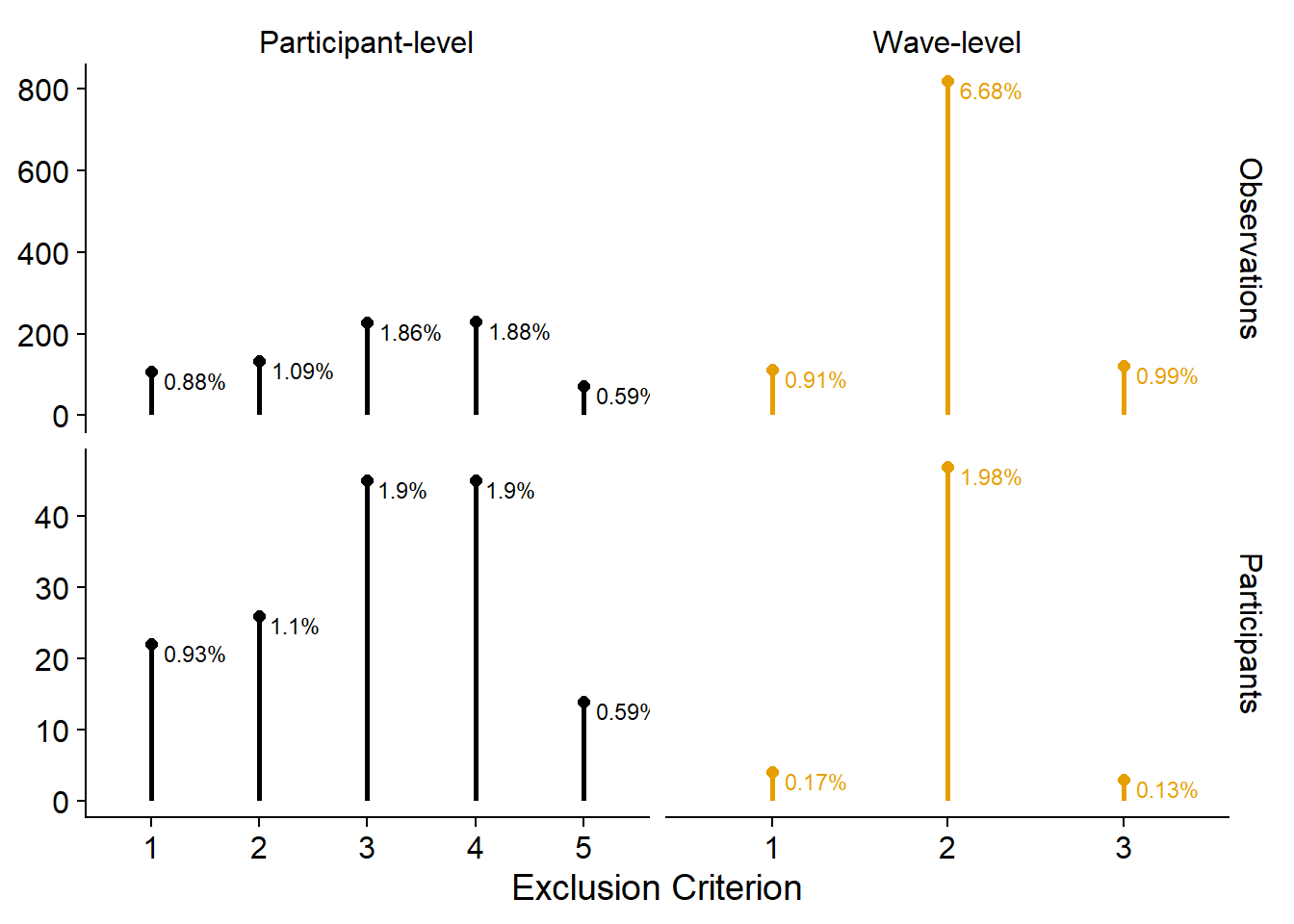Next, I plot the distributions of the use variables. I want to show distributions, but there are a couple of single large values, so violin or density plots will look strange with a long tail. Raincloud plots could solve that to a degree, but they duplicate information. I’ll go with a doptplot, namely beeswarm plots.

Like before, because I facet, I’ll turn the data into the long format first. I’m also not sure whether I like vertical or horizontal beeswarms, so I’ll do both.

``````# temporary data file in long format
dat <-
working_file %>%
pivot_longer(
contains("_time"), # only the time variables
names_to = "measure",
values_to = "Hours per day"
) %>%
mutate(
measure = as.factor(str_to_title(str_remove(measure, "_time"))), # prettier factor levels
measure = fct_recode(
measure,
"TV" = "Tv"
),
measure = fct_relevel(
measure,
c(
"Music",
"TV",
"Films",
"Games",
"Books",
"Magazines",
"Audiobooks",
"Total"
)
)
)

# summary stats for plotting means over time
dat_summary <-
dat %>%
group_by(wave, measure) %>%
summarise(
mean = mean(`Hours per day`, na.rm = T),
sd = sd(`Hours per day`, na.rm = T)
) %>%
mutate(
across(
mean:sd,
~ round(.x, digits = 1)
)
)

# let's try horizontal first (the commented out section adds a vertical line where the mean is)
ggplot(
dat,
aes(
x = `Hours per day`,
y = 1,
color = measure,
fill = measure
)
) +
geom_quasirandom(groupOnX=FALSE, size = 0.1, alpha = 0.5) +
# geom_vline(
#   data = dat_summary,
#   aes(
#     xintercept = mean,
#     color = measure
#   ),
#   linetype = "dashed"
# ) +
geom_point(
data = dat_summary,
aes(
x = mean,
y = 0.55
),
shape = 25,
size = 2
) +
facet_grid(measure ~ wave) +
geom_text(
data = dat_summary,
aes(
x = 20,
y = 1.4,
label = paste0("M = ", mean)
),
size = 3,
color = "black"
) +
geom_text(
data = dat_summary,
aes(
x = 19.6,
y = 1.3,
label = paste0("SD = ", sd)
),
size = 3,
color = "black"
) +
theme_cowplot() +
scale_colour_manual(values=cb_palette) +
scale_fill_manual(values = cb_palette) +
theme(
axis.text.y = element_blank(),
axis.title.y = element_blank(),
axis.ticks.y = element_blank(),
axis.line.y = element_blank(),
strip.background.x = element_blank(),
strip.background.y = element_blank(),
legend.position = "none"
) -> figure2.1

figure2.1``````I guess the trendline makes it easier to see developments (or lack thereof) over time.

``````ggplot(
dat,
aes(
x = as.factor(wave),
y = `Hours per day`,
color = measure,
fill = measure,
group = 1
)
) +
geom_quasirandom(size = 0.1, alpha = 0.2) +
geom_line(
data = dat_summary,
aes(
y = mean
),
size = 1
) +
facet_wrap(~ measure, ncol = 2) +
geom_text(
data = dat_summary,
aes(
x = as.factor(wave),
y = -1.2,
label = paste0("M = ", mean)
),
size = 2.5,
color = "black"
) +
geom_text(
data = dat_summary,
aes(
x = as.factor(wave),
y = -2.9,
label = paste0("SD = ", sd)
),
size = 2.5,
color = "black"
) +
theme_cowplot() +
scale_colour_manual(values=cb_palette) +
scale_fill_manual(values = cb_palette) +
xlab("Wave") +
theme(
strip.background.x = element_blank(),
strip.background.y = element_blank(),
legend.position = "none"
) -> figure2.2

figure2.2``````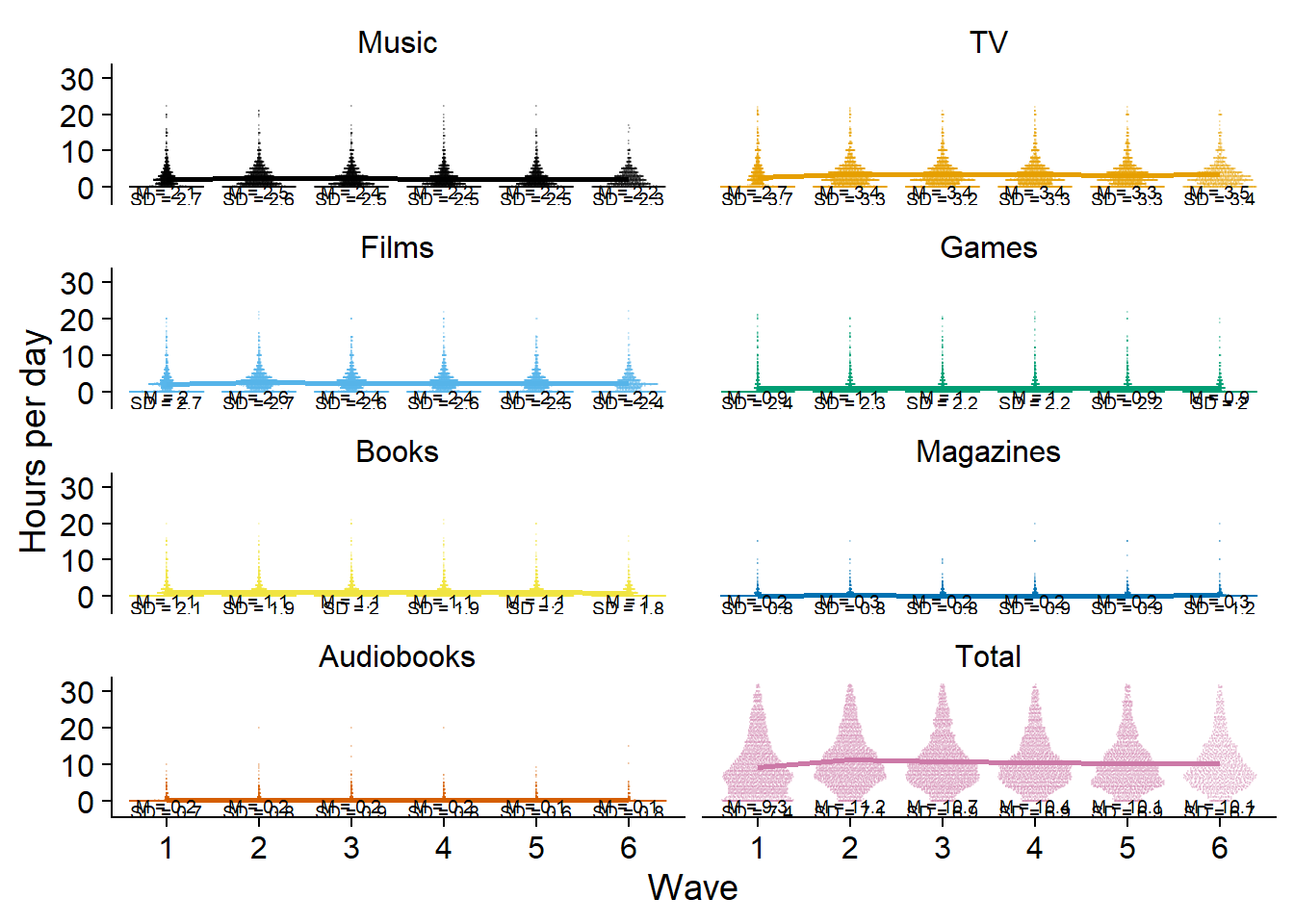Then again, for distributions with a small mean, the trendline blocks everything. Plus, because total time extends the y-axis, there’s lots of white space. I’ll give total time its separate plot to solve that issue. I also won’t have trendlines for the separate media, only for the total (too lazy to write the above into a function, so I’ll copy paste the code from above.).

``````ggplot(
dat %>%
filter(measure != "Total"),
aes(
x = as.factor(wave),
y = `Hours per day`,
color = measure,
fill = measure,
group = 1
)
) +
geom_quasirandom(size = 0.1, alpha = 0.2) +
# geom_line(
#   data = dat_summary,
#   aes(
#     y = mean
#   ),
#   size = 1
# ) +
facet_wrap(~ measure, ncol = 2) +
geom_text(
data = dat_summary %>%
filter(measure != "Total"),
aes(
x = as.factor(wave),
y = -1.2,
label = paste0("M = ", mean)
),
size = 2.5,
color = "black"
) +
geom_text(
data = dat_summary %>%
filter(measure != "Total"),
aes(
x = as.factor(wave),
y = -2.9,
label = paste0("SD = ", sd)
),
size = 2.5,
color = "black"
) +
theme_cowplot() +
scale_colour_manual(values=cb_palette) +
scale_fill_manual(values = cb_palette) +
xlab("Wave") +
theme(
strip.background.x = element_blank(),
strip.background.y = element_blank(),
legend.position = "none"
) -> figure2.3

figure2.3``````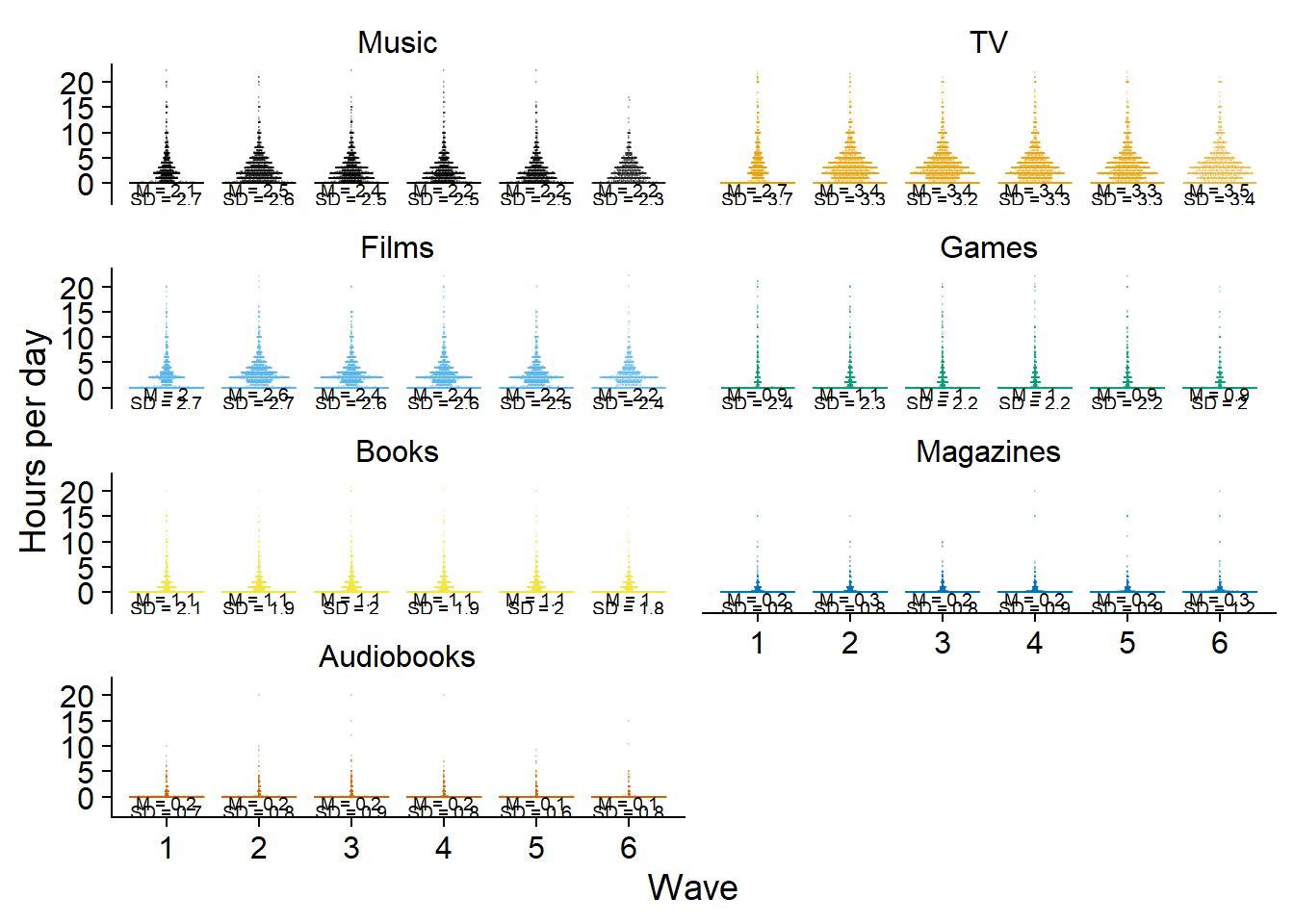``````ggsave(
here("figures", "figure2.png"),
plot = figure2.3,
width = 21 * 0.8,
height = 29.7 * 0.8,
units = "cm",
dpi = 300
)``````

Then a separate plot for total time.

``````ggplot(
dat %>%
filter(measure == "Total"),
aes(
x = as.factor(wave),
y = `Hours per day`,
group = 1
)
) +
geom_quasirandom(size = 0.1, alpha = 0.2, color = "#CC79A7") +
geom_line(
data = dat_summary %>%
filter(measure == "Total"),
aes(
y = mean
),
size = 0.5,
color = "#CC79A7"
) +
geom_text(
data = dat_summary %>%
filter(measure == "Total"),
aes(
x = as.factor(wave),
y = -1.2,
label = paste0("M = ", mean)
),
size = 3,
color = "black"
) +
geom_text(
data = dat_summary %>%
filter(measure == "Total"),
aes(
x = as.factor(wave),
y = -2.4,
label = paste0("SD = ", sd)
),
size = 3,
color = "black"
) +
theme_cowplot() +
xlab("Wave") +
ylab("Total hours per day") +
theme(
strip.background.x = element_blank(),
strip.background.y = element_blank(),
legend.position = "none"
) -> figure3

figure3``````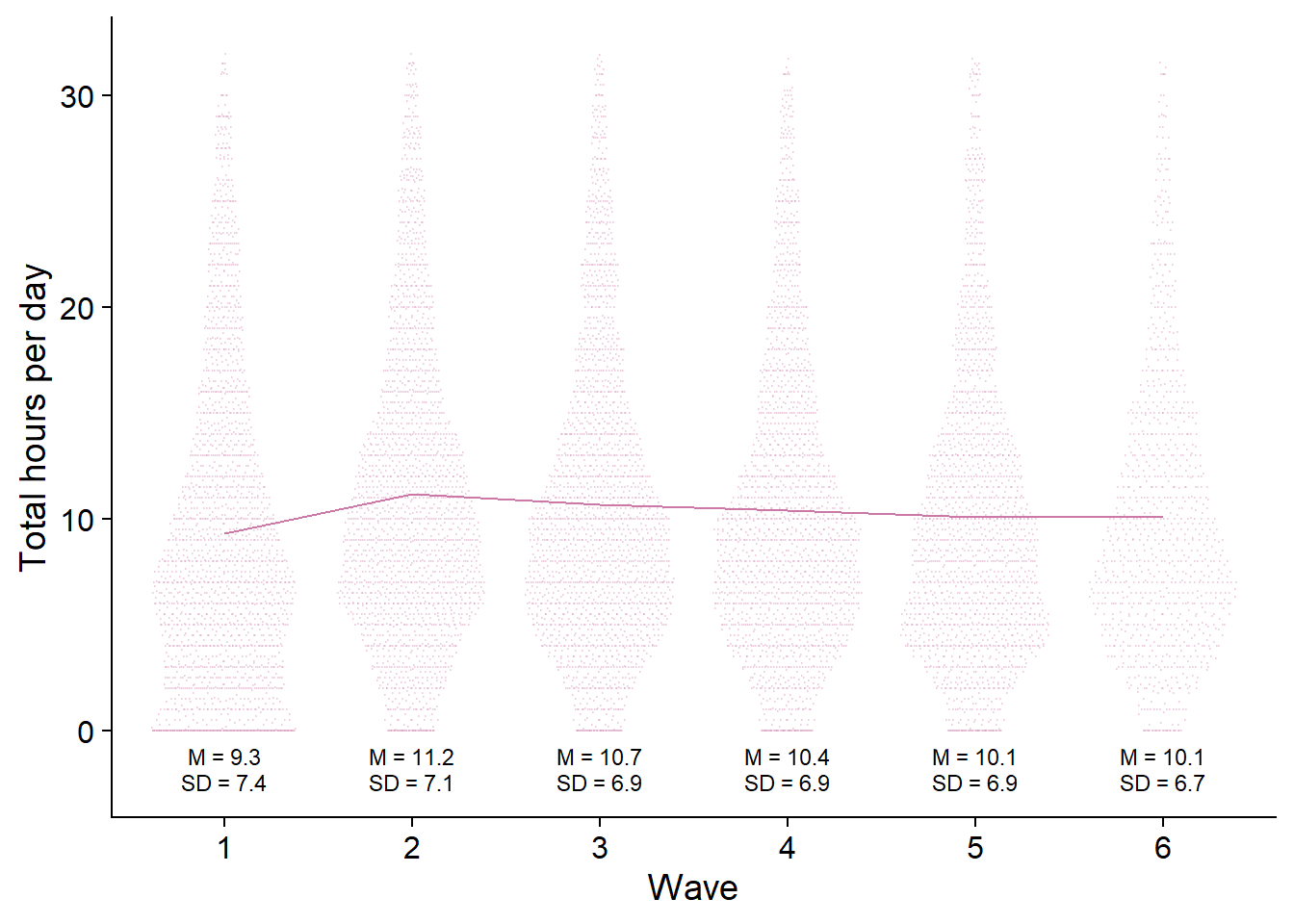``````ggsave(
here("figures", "figure3.png"),
plot = figure3,
width = 21 * 0.9,
height = 29.7 * 0.4,
units = "cm",
dpi = 300
)``````

Then, I need to think of a way to visualize use vs. nonuse over time. Bar graphs could work, but that would mean a lot of bar graphs (one per medium) per wave, which is probably overwhelming. Plus, if I show raw counts, it could be confusing as well, simply because height of the bar != number of participants. If everyone at wave 1 used only one medium, but at wave two everyone used all media, it’ll look like an increase in sample size.

So proportions make the most sense, simple and straightforward.

``````# get proportions of users per medium and wave
dat <-
working_file %>%
pivot_longer(
contains("_time"), # only the time variables
names_to = "measure",
values_to = "Hours per day"
) %>%
mutate(
# get use vs. no use in long format
used = if_else(`Hours per day` == 0, 0, 1)
) %>%
# no need for total time
filter(used == 1, measure != "total_time") %>%
# remove "tome appendix
mutate(measure = as.factor(str_to_title(str_remove(measure, "_time")))) %>%
# count how often a medium was used
count(wave, measure, used) %>%
# add total N per wave (of only those that will be included in analysis)
left_join(
.,
working_file %>%
count(wave, name = "N")
) %>%
# get proprotion
mutate(
proportion = round(n / N, digits = 2) * 100,
measure = fct_recode(measure, "TV" = "Tv"),
measure = fct_relevel(
measure,
c(
"Music",
"TV",
"Films",
"Games",
"Books",
"Magazines",
"Audiobooks"
)
)
)

ggplot(
dat,
aes(
x = as.factor(wave),
y = proportion,
group = measure,
color = measure
)
) +
geom_point(size = 3) +
geom_line(size = 1) +
scale_colour_manual(values=cb_palette) +
scale_fill_manual(values = cb_palette) +
ylab("% of people who used medium") +
xlab("Wave") +
geom_dl(aes(label = measure), method = list(dl.trans(x = x + 0.25), "last.points", cex = 0.6)) +
theme(
legend.position = "none"
) -> figure4

figure4``````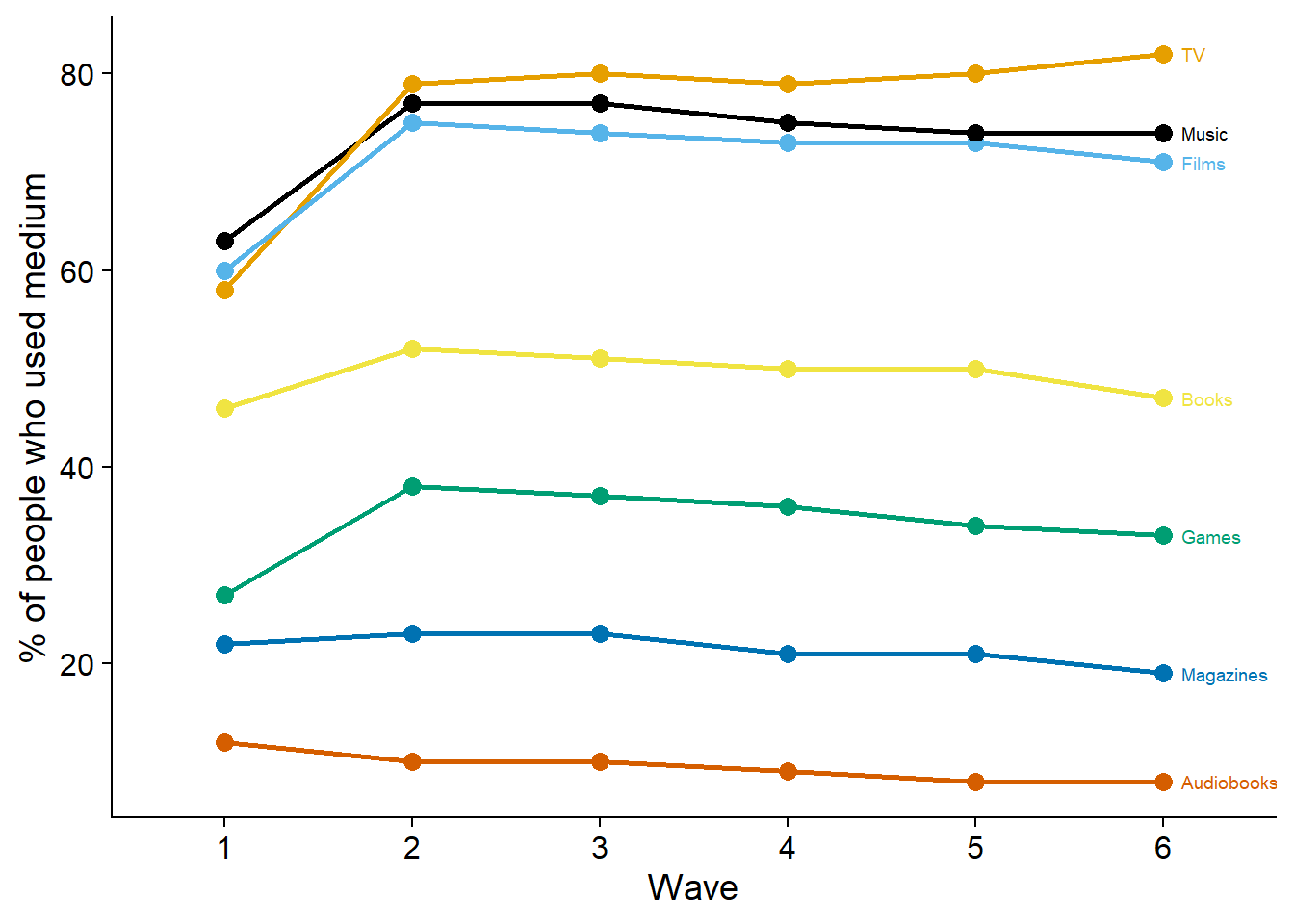``````ggsave(
here("figures", "figure4.png"),
plot = figure4,
width = 21 * 0.9,
height = 29.7 * 0.4,
units = "cm",
dpi = 300
)``````

Next, I’ll do a beeswarm plot for well-being.

``````# temporary data file in long format
dat <-
working_file %>%
pivot_longer(
c(affect, life_satisfaction), # only the aggregated well-being variables
names_to = "measure",
values_to = "Value"
) %>%
mutate(
measure = fct_recode(
as.factor(measure),
"Affect" = "affect",
"Life satisfaction" = "life_satisfaction"
)
)

# summary stats for plotting means over time
dat_summary <-
dat %>%
group_by(wave, measure) %>%
summarise(
mean = mean(Value, na.rm = T),
sd = sd(Value, na.rm = T)
) %>%
mutate(
across(
mean:sd,
~ round(.x, digits = 1)
)
)

ggplot(
dat,
aes(
x = as.factor(wave),
y = Value,
color = measure,
fill = measure,
group = 1
)
) +
geom_quasirandom(size = 0.1, alpha = 0.2) +
geom_line(
data = dat_summary,
aes(
y = mean
),
size = 0.5
) +
facet_wrap(~ measure, ncol = 2) +
geom_text(
data = dat_summary,
aes(
x = as.factor(wave),
y = -0.5,
label = paste0("M = ", mean)
),
size = 2.5,
color = "black"
) +
geom_text(
data = dat_summary,
aes(
x = as.factor(wave),
y = -0.9,
label = paste0("SD = ", sd)
),
size = 2.5,
color = "black"
) +
theme_cowplot() +
scale_colour_manual(values=c("#E69F00", "#000000")) +
scale_fill_manual(values = c("#E69F00", "#000000")) +
xlab("Wave") +
theme(
axis.title.y = element_blank(),
strip.background.x = element_blank(),
strip.background.y = element_blank(),
legend.position = "none"
) -> figure5

figure5``````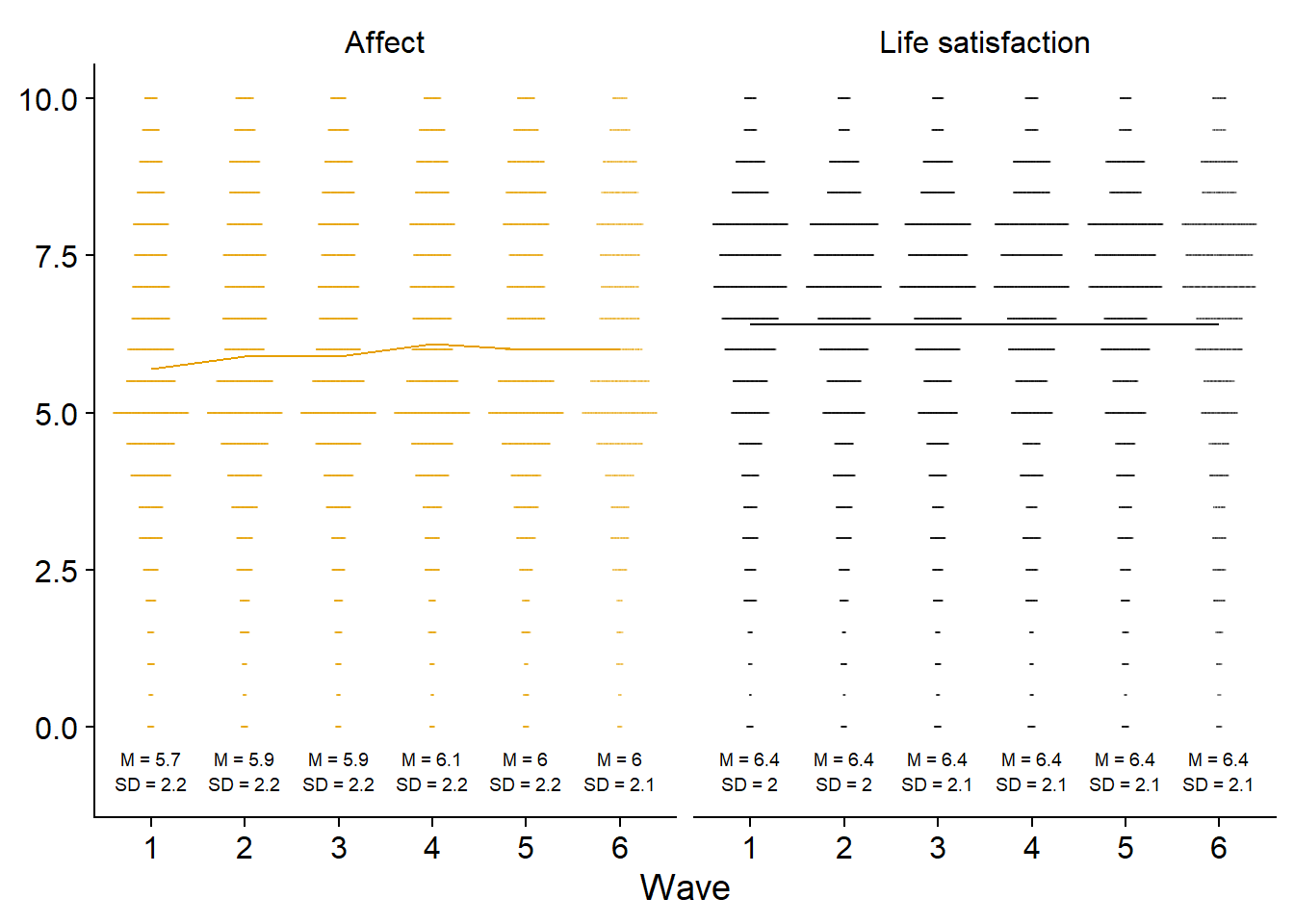``````ggsave(
here("figures", "figure5.png"),
plot = figure5,
width = 21 * 0.8,
height = 29.7 * 0.4,
units = "cm",
dpi = 300
)``````